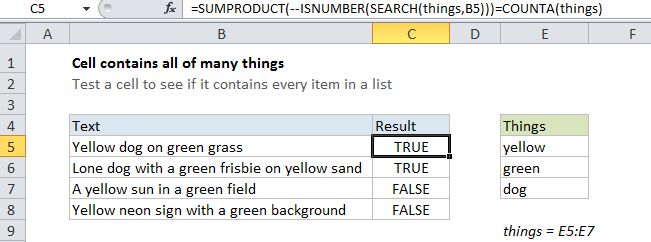How to check if cell contains all of many things in Excel

If you want to test a cell to see if it contains all items in a list, you can do so with a formula that uses the SEARCH function, with help from the ISNUMBER, SUMPRODUCT, and COUNTA functions.

Case study: Let’s say you have a list of text strings in the range B5:B8, and you want to find out if these cells contain all of the words in another range, E5:E7.

You could build a formula that uses nested IF statements to check for each item, but that won’t scale well if you have a lot of things to look for.  Each time you add an word to look for you’ll need to add another nested IF and adjust parentheses.Explanation

Solution

The solution is to to create a formula counts all matches at once. Once we have that, we simply compare that count with the count of items we’re looking for. If they match, we know a cell contains all items.

In the example shown, the formula we’re using is:

`=SUMPRODUCT(--ISNUMBER(SEARCH(things,B5)))=COUNTA(things)`

How this formula works

The key is this snippet:

`ISNUMBER(SEARCH(things,B5)`

This is based on another formula (explained in detail here) that simply checks a cell for a single substring. If the cell contains the substring, the formula returns TRUE. If not, the formula returns FALSE.

Also See:   Count cells equal to case sensitive in Excel

However, if we give the same formula a list of things (in this case, we are using a named range called “things”, E5:E7) it will give us back a list of TRUE / FALSE values, one for each item in . The result is an array that looks like this:

{TRUE;TRUE;TRUE}

Where each TRUE represents a found item, and each FALSE represents an item not found.

We can force the TRUE / FALSE values to 1s and 0s with a double negative (–, also called a double unary):

`--ISNUMBER(SEARCH(things,B5))`

which yields an array like this:

{1;1;1}

Next, we process this array with SUMPRODUCT, which will give us a total sum. If this sum is equal to the number of items in the named range “things”, we know we’ve found all things and can return TRUE. The way we do this is to compare the two numbers directly. We get a count of non-blank cells in “things” using COUNTA:

`=COUNTA(things)`

With a hard-coded list

There’s no requirement that you use a range for your list of things. If you’re only looking for a small number of things, you can use a list in array format, which is called an array constant. For example, if you’re just looking for the colors red, blue, and green, you can use {“red”,”blue”,”green”} like this:

`=SUMPRODUCT(--ISNUMBER(SEARCH({"yellow","green","dog"},B5)))=COUNTA(things)`